# 现实比理论要复杂

2019/04/10 10:10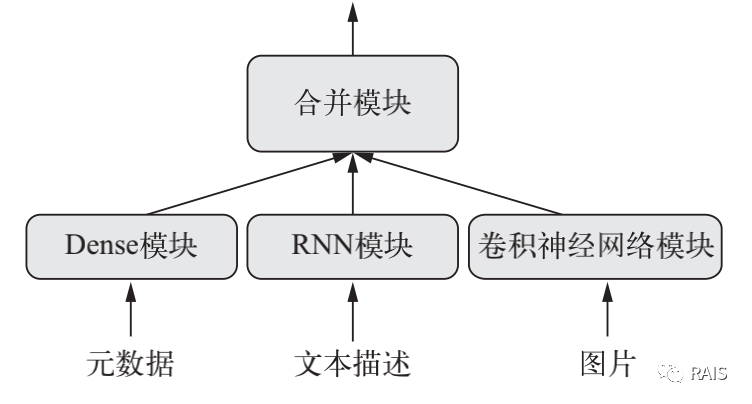### 函数式 API

def run():
# 我们学过的用 Sequential 定义层
seq_model = Sequential()
seq_model.summary()
​
# 用函数的方式定义层
input_tensor = Input(shape=(64,))
x = layers.Dense(32, activation='relu')(input_tensor)
x = layers.Dense(32, activation='relu')(x)
output_tensor = layers.Dense(10, activation='softmax')(x)
model = Model(input_tensor, output_tensor)
model.summary()


### 多输入模型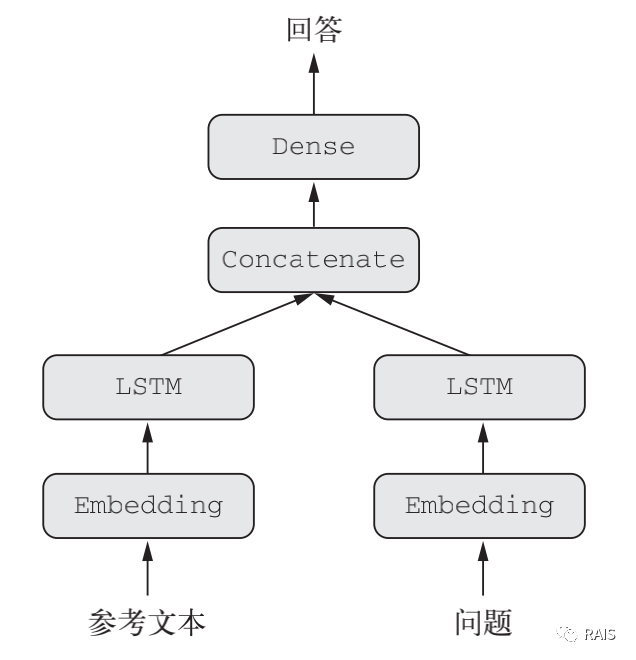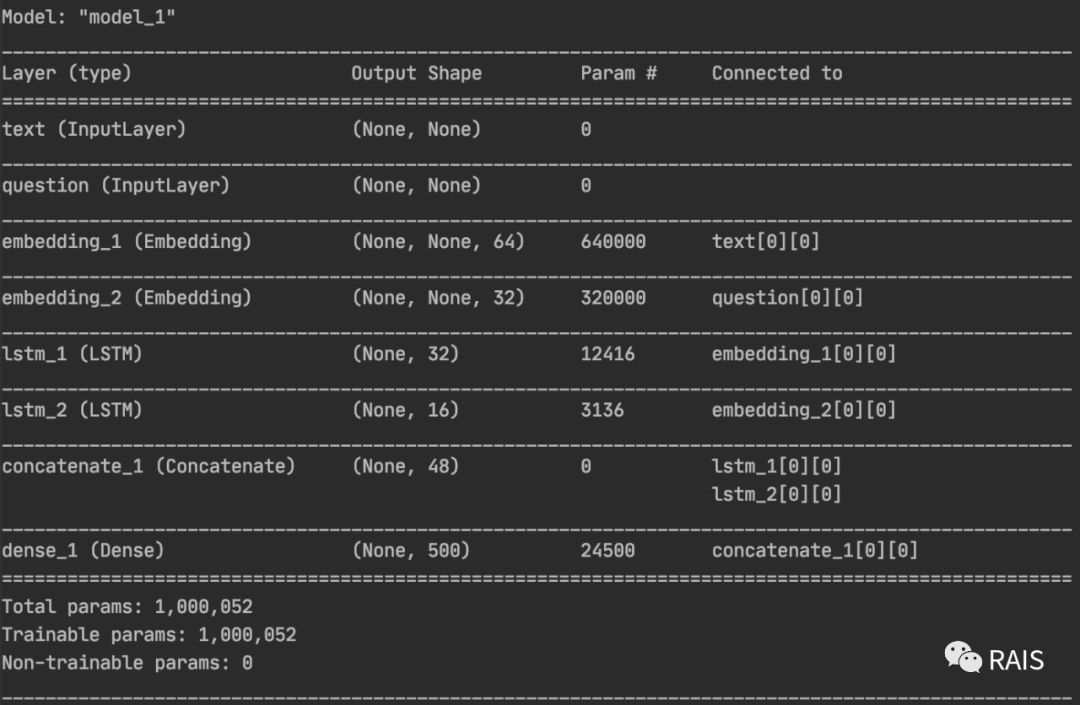#!/usr/bin/env python3
​
import time
​
import keras
​import numpy as np
from keras import Input
from keras import layers
from keras.models import Model
​
​
def run():
text_vocabulary_size = 10000
question_vocabulary_size = 10000
# 文本输入
text_input = Input(shape=(None,), dtype='int32', name='text')
# 将输入转换为向量
embedded_text = layers.Embedding(text_vocabulary_size, 64)(text_input)
# 将输入转换为单个向量
encoded_text = layers.LSTM(32)(embedded_text)
# 对问题进行如上处理
question_input = Input(shape=(None,), dtype='int32', name='question')
embedded_question = layers.Embedding(question_vocabulary_size, 32)(question_input)
encoded_question = layers.LSTM(16)(embedded_question)
​
# 将问题和文本联系起来
concatenated = layers.concatenate([encoded_text, encoded_question], axis=-1)
# 添加一个 softmax 分类器
# 构建模型
model = Model([text_input, question_input], answer)
model.summary()
​
model.compile(optimizer='rmsprop', loss='categorical_crossentropy', metrics=['acc'])
​
# 生成虚拟训练数据，参考意义不大
num_samples = 1000
max_length = 100
text = np.random.randint(1, text_vocabulary_size, size=(num_samples, max_length))
question = np.random.randint(1, question_vocabulary_size, size=(num_samples, max_length))
model.fit([text, question], answers, epochs=10, batch_size=128)
model.fit({'text': text, 'question': question}, answers, epochs=10, batch_size=128)
​
​
if __name__ == "__main__":
time_start = time.time()
run()
time_end = time.time()
print('Time Used: ', time_end - time_start)


### 多输出模型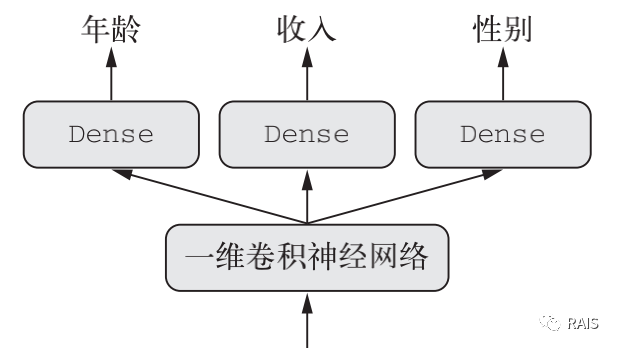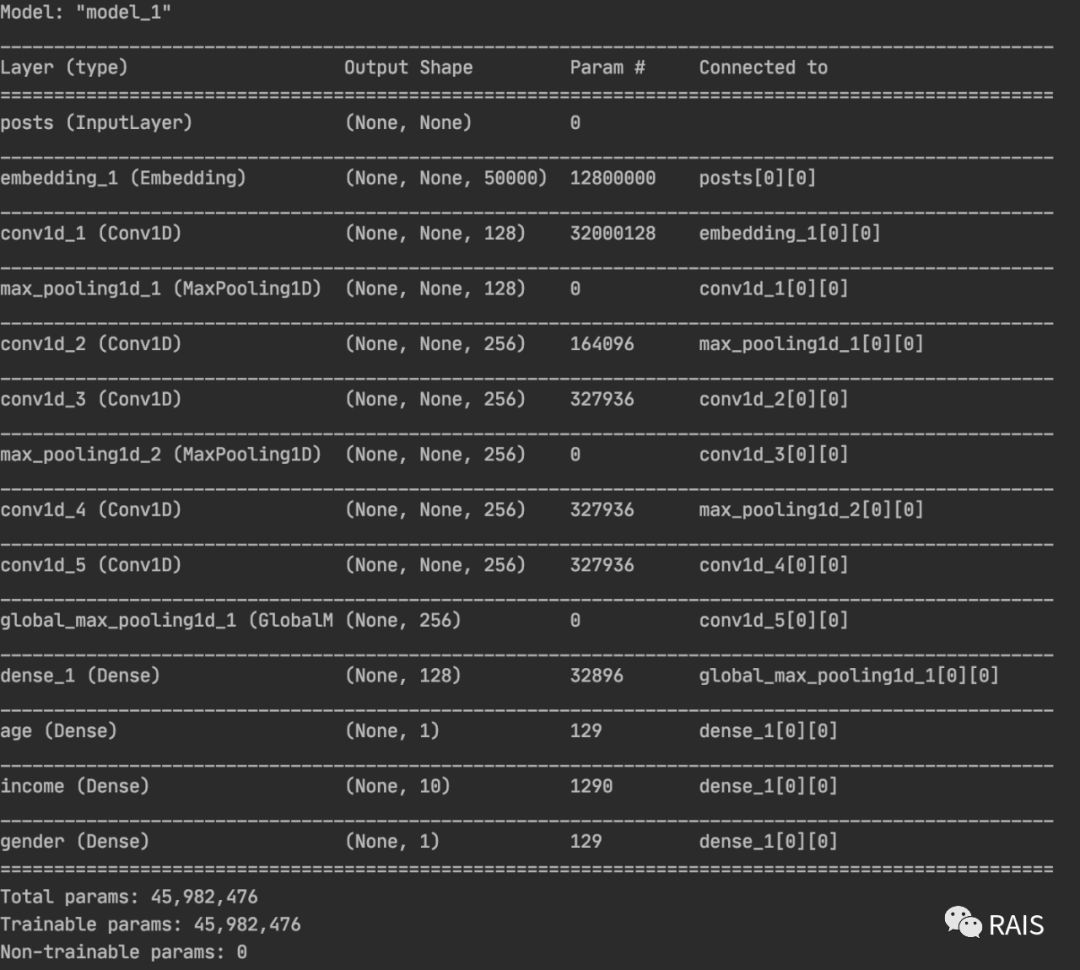def run():
vocabulary_size = 50000
num_income_groups = 10
posts_input = Input(shape=(None,), dtype='int32', name='posts')
embedded_posts = layers.Embedding(256, vocabulary_size)(posts_input)
x = layers.Conv1D(128, 5, activation='relu')(embedded_posts)
x = layers.MaxPooling1D(5)(x)
x = layers.Conv1D(256, 5, activation='relu')(x)
x = layers.Conv1D(256, 5, activation='relu')(x)
x = layers.MaxPooling1D(5)(x)
x = layers.Conv1D(256, 5, activation='relu')(x)
x = layers.Conv1D(256, 5, activation='relu')(x)
x = layers.GlobalMaxPooling1D()(x)
x = layers.Dense(128, activation='relu')(x)
age_prediction = layers.Dense(1, name='age')(x)
income_prediction = layers.Dense(num_income_groups, activation='softmax', name='income')(x)
gender_prediction = layers.Dense(1, activation='sigmoid', name='gender')(x)
model = Model(posts_input, [age_prediction, income_prediction, gender_prediction])
model.summary()

# 关键在这里
model.compile(optimizer='rmsprop',
loss={'age': 'mse',
'income': 'categorical_crossentropy',
'gender': 'binary_crossentropy'},
loss_weights={'age': 0.25,
'income': 1.,
'gender': 10.})
# model.fit(posts, [age_targets, income_targets, gender_targets], epochs=10, batch_size=64)


### 图结构

#### Inception 模块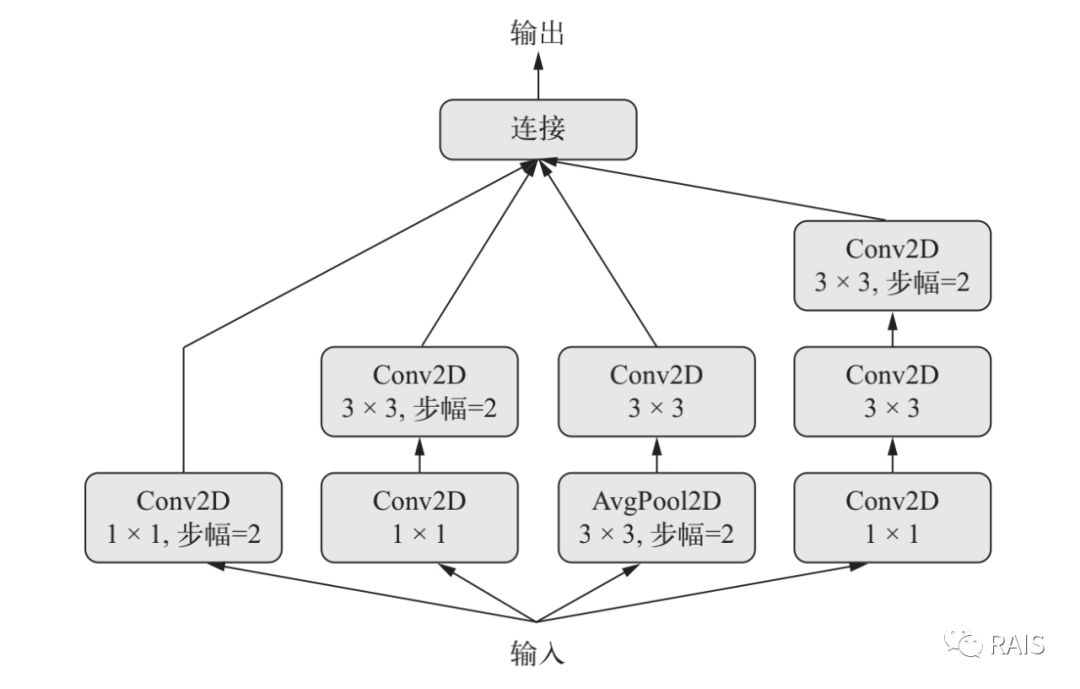branch_a = layers.Conv2D(128, 1, activation='relu', strides=2)(x)
branch_b = layers.Conv2D(128, 1, activation='relu')(x)
branch_b = layers.Conv2D(128, 3, activation='relu', strides=2)(branch_b)
branch_c = layers.AveragePooling2D(3, strides=2)(x)
branch_c = layers.Conv2D(128, 3, activation='relu')(branch_c)
branch_d = layers.Conv2D(128, 1, activation='relu')(x)
branch_d = layers.Conv2D(128, 3, activation='relu')(branch_d)
branch_d = layers.Conv2D(128, 3, activation='relu', strides=2)(branch_d)
output = layers.concatenate([branch_a, branch_b, branch_c, branch_d], axis=-1)


#### 残差连接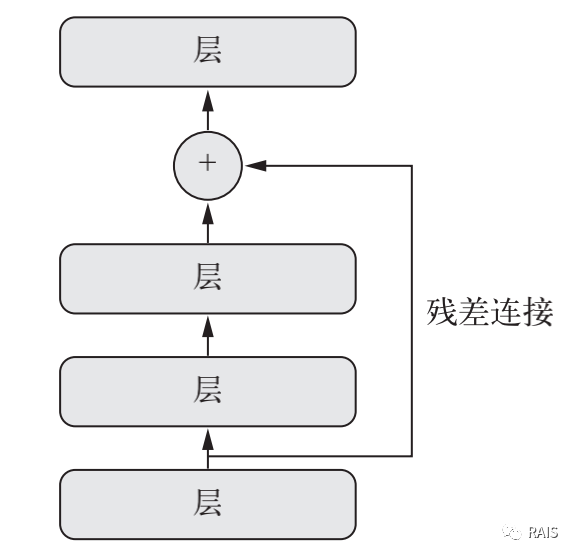x = ...
y = layers.Conv2D(128, 3, activation='relu', padding='same')(x)
y = layers.Conv2D(128, 3, activation='relu', padding='same')(y)
y = layers.Conv2D(128, 3, activation='relu', padding='same')(y)
y = layers.add([y, x])


### 共享层权重

# 定义一次
lstm = layers.LSTM(32)
left_input = Input(shape=(None, 128))
# 使用
left_output = lstm(left_input)
right_input = Input(shape=(None, 128))
# 使用
right_output = lstm(right_input)
merged = layers.concatenate([left_output, right_output], axis=-1)
predictions = layers.Dense(1, activation='sigmoid')(merged)
model = Model([left_input, right_input], predictions)


# Xception 是一个网络
xception_base = applications.Xception(weights=None, include_top=False)
left_input = Input(shape=(250, 250, 3))
​right_input = Input(shape=(250, 250, 3))
left_features = xception_base(left_input)
right_input = xception_base(right_input)
merged_features = layers.concatenate([left_features, right_input], axis=-1)


### 总结

• 本文首发自公众号：RAIS，欢迎关注！### 作者的其它热门文章

0
0 收藏

0 评论
0 收藏
0